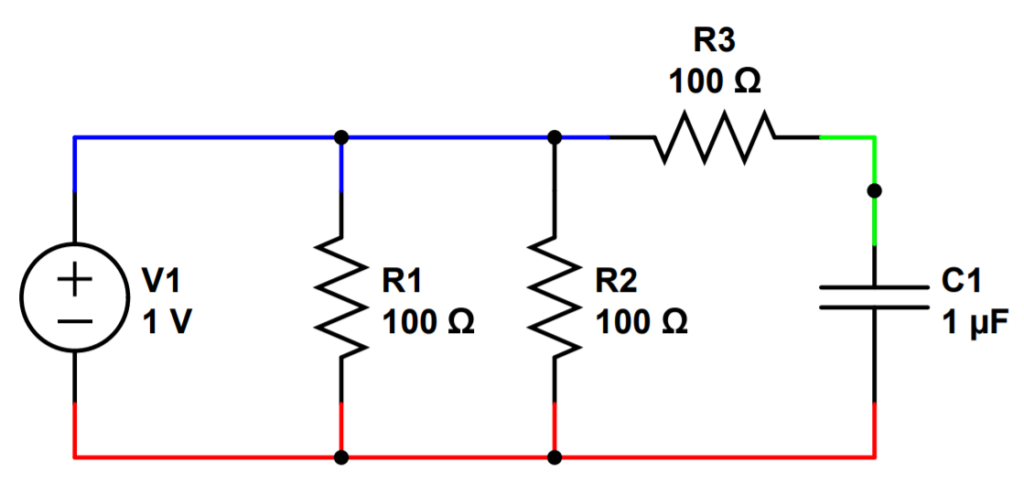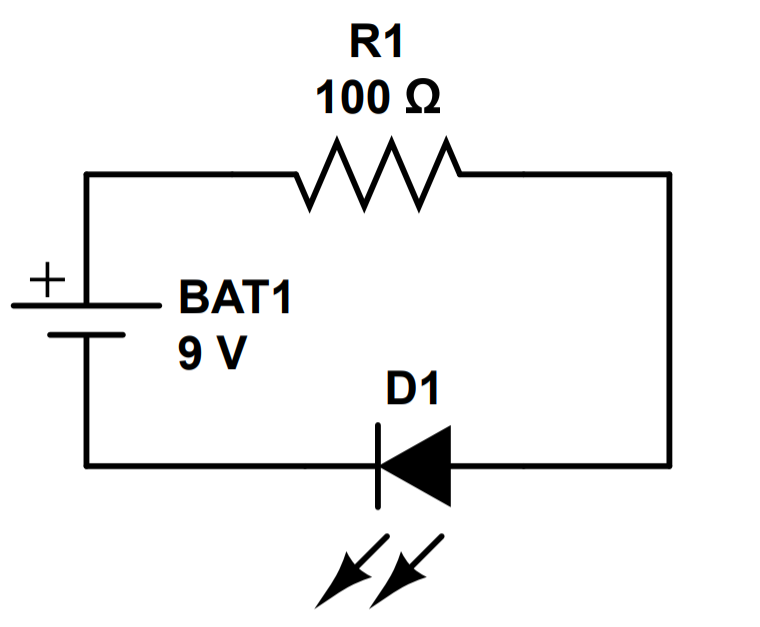# Direct Current Circuits

Direct Current (DC) is a one-directional flow of electric current. Direct current circuits are a type of electric circuit in which current flows in only one direction.

DC circuits are incredibly important to our lives. Most electronics and logic-based systems (i.e. computers) use DC. Everything that has a battery uses DC; laptops, cell phones, flashlights all use DC. Even cars use DC (supplied by a car battery). Desktop computers have power supplies to convert alternating current (AC) from a wall outlet into DC.

Learning about DC circuits is a great way to begin to understand electric circuits and electronics. That’s because DC is the system used in most electronics. Direct current systems are also easier to understand than systems using alternating current (AC) but AC systems rely on many of the same principles and circuit components as DC systems. We can learn about important principles with DC circuits and then use what we’ve learned to analyze circuits with other types of current.

That’s why DC circuits are the best way to learn about the three components that are considered the building blocks of classical electrical engineering: resistors, capacitors, and inductors.A DC circuit, showing a 1V source, three resistors and a capacitor. Each circuit node has a different color (blue, green, red).

DC Circuits are the basic building block of many electronics, and are the best way to begin to understand electrical circuits. Most electronics are DC and others use a DC power supply, so DC circuits is a topic that is of great importance.

There are two ‘tracks’ of knowledge when learning about any circuits; theoretical and practical. For electronics, there are fundamental (theoretical) principles, as well as components that function by making practical use of the theory.

For example, resistors are circuit components that rely on the principle of electrical resistance in order to function. It’s important to understand both in order to have a well rounded approach to circuits utilizing resistors.

## What are DC Circuits?

Let’s start by defining an electric circuit, also called an electric network. A simple circuit can be described as a closed loop connected to a power source. The power source provides a voltage (electric potential) that pushes electrons around the loop.

The electrons flow through the whole circuit, which is usually made of conductors and devices like resistors, capacitors, or inductors.

Electron flow is called electric current. Electrons actually flow in the opposite direction of current, and we usually think about current flow, not electron flow.

This is where the name Direct Current (DC) comes from. A direct current circuit is one in which the current only flows in one direction.

In other words in a DC circuit, the flow of electricity goes in one direction; from the positive terminal to the negative terminal. In a DC circuit, the value of the voltage might change. For instance, the voltage across the circuit might alternate from .5 to 1.5 volts. But the polarity of voltage, or direction of current flow never changes; it always goes in the same direction.

To put this into perspective, AC circuits feature an Alternating Current, in which the direction of current switches from one side to the other. Direct Current (DC) always pushes the electrons along in the same direction, but alternating current (AC) pushes electrons one way and then pulls them back in the other direction. We’ll explore more about AC circuits in the next module. For now just keep in mind that in a DC circuit, the current always flows in one direction; in an AC circuit the current alternates and flows back and forth within a circuit.

In order for electricity to flow through a circuit, it must have somewhere to come from and somewhere to go. If a circuit is connected to a power source but doesn’t give the electrons somewhere to go, electricity cannot flow. For instance, a wire in the circuit is no longer making contact on one side. This is called an open circuit. Open circuits are otherwise complete circuits that have a break somewhere, preventing current from flowing. Open circuits aren’t really circuits at all because current cannot flow within them. However, the term ‘open circuit’ describes a condition in which current would flow through the circuit.

## How do DC Circuits Work?

DC Circuits are made up of two main things: components, and conductors.

1. Components are the ‘blocks’ that make up the circuit. Power sources, resistors, capacitors, LEDs, and transistors are examples of common components.
2. Conductors provide paths for current between all of the components of the circuit.

### Components

Perhaps the most important component is the power source. Without a power source, there would be nothing to drive current in the circuit at all.

DC circuits are powered by direct current sources, which are power sources that generate a potential difference (voltage) between two points.

If we were to separate some electrons from their atoms and then connect the electrons, through conductors, back to their original atoms, we would have a DC circuit. The separation between the negatively charged electrons and positively charged atoms (ions) results in a difference of electric potential (voltage). Which is almost exactly what batteries do.

Batteries are common DC sources, generating a voltage that is close to their stated voltage, at least until they begin to be depleted. For example, a common battery in the US is a 1.5V ‘AA’ battery, which can be used to power a DC circuit. When you put batteries back to back (as in many devices like remote controls), you add the voltage of each one to the total. The total voltage represents the ‘push’ against the electrons in the circuit, like pressure in a pipe.

Another common component is a resistor, which is a device that restricts the flow of current. Why would we ever want to do that?

Let’s say we want to use a battery to power a DC circuit. We’ll want to include at least one resistor so the battery doesn’t short circuit! We connect the positive and negative terminals of the battery to each end of the resistor.

It also helps to have a visual confirmation that current is flowing. We can connect an LED in to the circuit, which will light up when current is flowing. Make sure that the negative terminal of the LED is connected to the negative terminal of the battery. The schematic of our circuit will look like this:A DC circuit consisting of a battery, resistor, and LED.

The battery’s voltage will push electrons electrons out of the negative terminal, through the resistor, and into the positive terminal of the battery. As the electrons flow, they generate current in the opposite direction.

The battery has an excess negative charge available inside it’s negative terminal, and positive ions that are trying to attract electrons in it’s positive terminal. Between the negative and positive terminals is a force that pushes electrons from the negative to the positive terminals if we connect them to each other. This force is what drives a DC circuit.

### Conductors

Circuits need something to connect the different components together. This is done with conductors, which are often copper or aluminum wires. The most important thing about conductors is that they allow current to flow with minimal resistance. Resistance creates a power loss so it is always best to minimize it whenever it is not wanted.

Over short distances, aluminum or copper provide a low enough resistance for circuits to function well.

Combined with the circuit components, conductors provide a complete path for electric current to follow through the circuit.

## Different Types of Current

This single direction movement is shown in the following chart. DC current is shown in red, and AC is in green. Other types of currents include pulses (in blue), and variable, which is another word for changing.

This module is focused on direct current (DC), shown in red.

As shown, direct current does not change with time. It is a flat line that does not go up or down. This means that the movement of electrons doesn’t speed up or slow down. The current doesn’t change direction, either. The electrons leave the power source, go through the circuit, and go back into the power source on the other terminal. Direct current means that the current doesn’t change.

In contrast, other types of current do change with time. A pulsating current changes intensity, but not direction. An alternating current, on the other hand, changes both intensity and direction. When the green plot of the alternating current crosses the x-axis (for time), it goes from a positive current, to zero, to negative current. This means that the current actually changes direction and the electrons flow in the opposite direction, until the current reverses again. We’ll go over this in greater detail in the next module, which will focus on AC current.

In the above example, the current never, ever changes. In real life, this isn’t possible; there is always some variation (however small) in the current supplied by a man-made machine. The definition of a DC circuit is one in which the current changes direction. It may shift values, but it is always positive (a negative value indicates a current moving in the opposite direction).

## How are DC Sources Powered?

In general, we will use a battery as our source of electrical energy for DC circuits, and we’ll cover batteries in the next lesson. A battery can be thought of as a pump for electric charge, as it pushes electrons through a circuit via its’ negative terminal, and pulls electrons into its’ positive terminal. In doing so, it powers the circuit to function; without a battery or other power source, an electric circuit would just be a bunch of connected devices that would like a pipes containing water but without a pump to circulate or push the water.

However, the type of source does not actually matter. It could be a battery, battery array, power supply, or even something called a function generator. Function generators are machines that can produce a wide range of voltage sources to help us build and test circuits.

A DC circuit generally contains at least one power source and at least one resistor, and this is the simple system that we will use to begin our exploration of electrical circuits. If you can understand such a system, you will have an intuitive knowledge of what a circuit is and important concepts like current, voltage, and resistance, and Ohm’s Law. Along the way we’ll learn the basics of electrical components like batteries and resistors. We’ll discuss resistors in series and parallel, voltage dividers, Kirchoff’s Current and Voltage Laws, combinations of resistors and capacitors, and finish this module by learning about a really cool circuit, the RC circuit. If you can understand the RC circuit, you’ll be well on your way to a mastery of basic electrical theory!

Let’s continue on to Lesson 2: Series and Parallel Circuits!

Module 3: DC Circuits

Lesson 0: Introduction to Module 3

Lesson 1: Introduction to DC Circuits

Lesson 2: Series and Parallel Circuits

Lesson 3: DC Power Sources and Batteries

Lesson 4: Resistors, Capacitors, and Inductors

Lesson 5: Resistors in Series

Lesson 6: Resistors in Parallel

Lesson 7: Voltage Dividers

Lesson 8: Kirchoff’s Current Law

Lesson 9: Kirchoff’s Voltage Law

Lesson 10: Capacitors

Lesson 11: Dielectric Materials

Lesson 12: Capacitors in Parallel

Lesson 13: Capacitors in Series

Lesson 14: Capacitors in Series and Parallel